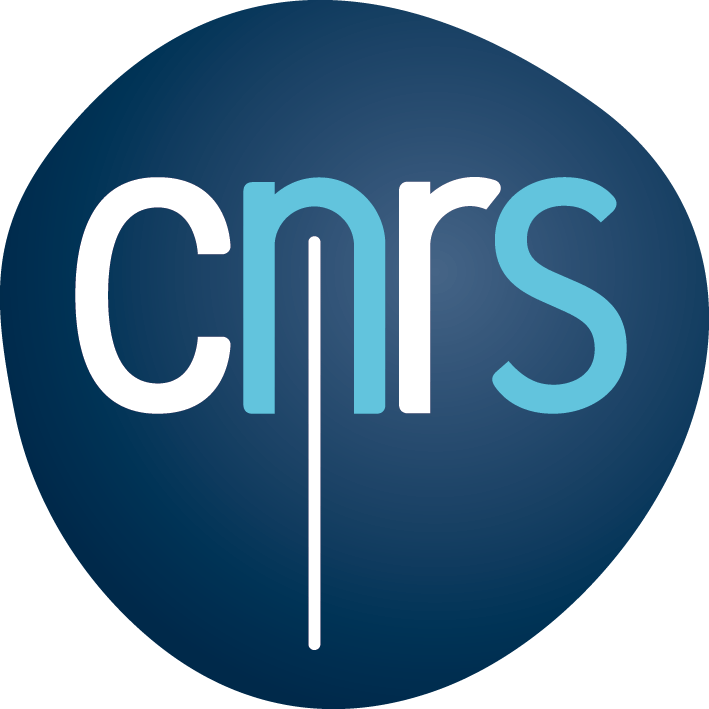We offer a very simple and convenient interface for integrating new benchmark problems/functions. First, you could define a new test_problem as you like. Note that the <vector> header is already imported in “ioh.hpp”.

#include "ioh.hpp"

std::vector<double> test_problem(const std::vector<double> &)
{
// the actual function body start here
// ...
}


Then, you only need to “wrap” this new function as follows:

auto new_problem = ioh::problem::wrap_function<double>(
&test_problem,
"test_problem" // name for the new function
);
std::cout << new_problem.meta_data() << std::endl;


After wrapping, we could also create this test_problem from the problem factory. Note that, the instance id is ineffective in this approach since we haven’t implemented it for the wrapped problem.

auto &factory = ioh::problem::ProblemRegistry<ioh::problem::Real>::instance();
auto new_problem_f = factory.create(
"test_problem",  // create by name
1,               // instance id
10               // number of search variables
);


Alternatively, one might wish to create the new problem by subclassing the abstract problem class in IOHexperimenter, taking benefits of implementing more details, e.g., aforementioned transformations. This can be done by inheriting the corresponding problem registration class, which is

• ioh::problem::IntegerProblem for pseudo-Boolean problems, and
• ioh::problem::RealProblem for continuous problems.

In the below example, we show how to do this for pseudo-Boolean problems.

class NewBooleanProblem final : public ioh::problem::IntegerProblem<NewBooleanProblem>
{
protected:
// [mandatory] The evaluate method is mandatory to implement
std::vector<int> evaluate(const std::vector<int> &x) override
{
// the function body goes here
}

// [optional] If one wish to implement transformations on objective values
std::vector<double> transform_objectives(std::vector<double> y) override
{

}

// [optional] If one wish to implement transformations on search variables
std::vector<double> transform_objectives(std::vector<double> y) override
{

}

public:
/// [mandatory] This constructor always take instance as input even
/// if it is ineffective by default. instance would be effective if and only if
/// at least one of transform_objectives and transform_objectives is implemented
NewBooleanProblem(const int instance, const int n_variables) :
IntegerProblem(
1,                     // problem id, which will be overwritten when registering this class in all pseudo-Boolean problems
instance,              // the instance id
"NewBooleanProblem",   // problem name
n_variables,           // search dimensionality
1,                     // number of objectives, only support 1 for now
ioh::common::OptimizationType::Minimization
)
)
{
}
};


We offer a simple base class for customized loggers. You can define a new logger class inheriting the logger class in base.hpp.

First, you need to define a new track_problem function to access the metadata of the problem to be tested. The track_problem function will be revoked in the attach_logger function of the problem class. You should also define a track_suite function for tracking information of suites for your experiment.

void track_problem(const problem::MetaData& problem)
{

}

void track_suite(const std::string& suite_name)
{

}



Then you should define a log function performing logging operations. The log function will be revoked in the evaluate function of the problem class. The LogInfo class provides variables of the optimization state.

void log(const LogInfo& log_info)
{

}

struct LogInfo
{
// The number of evaluations that have been used.
size_t evaluations;

// The fitness values of the best-so-far solution calculating without applying transformations.
double y_best;

// The fitness value of the last evaluated solution.
double transformed_y;

// The fitness value of the best-so-far solution.
double transformed_y_best;

// The last evaluated solution.
problem::Solution<double> current;

// The optimum if it exists.
problem::Solution<double> objective;
};



Also, a flush functions is required to close the logger at last.

void flush()
{

}


Please check this example for recording evaluation information during the optimization process into csv files.